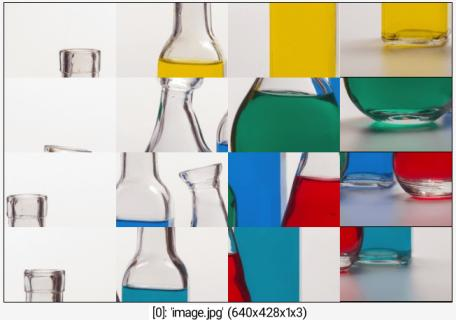Reference

 Table of Contents  ▸  List of Commands  ▸  Geometry Manipulation  ▸  append_tiles ◀  append    |    apply_scales  ▶

# append_tiles

## Arguments:

• _M>=0,_N>=0,0<=_centering_x<=1,0<=_centering_y<=1

## Description:

Append MxN selected tiles as new images.

If N is set to 0, number of rows is estimated automatically.
If M is set to 0, number of columns is estimated automatically.
If M and N are both set to 0, auto-mode is used.
If M or N is set to 0, only a single image is produced.
centering_x and centering_y tells about the centering of tiles when they have different sizes.

## Default values:

M=0, N=0, centering_x=centering_y=0.5.

## Example of use:

\$ gmic image.jpg split xy,4 append_tiles ,Command: image.jpg split xy,4 append_tiles ,